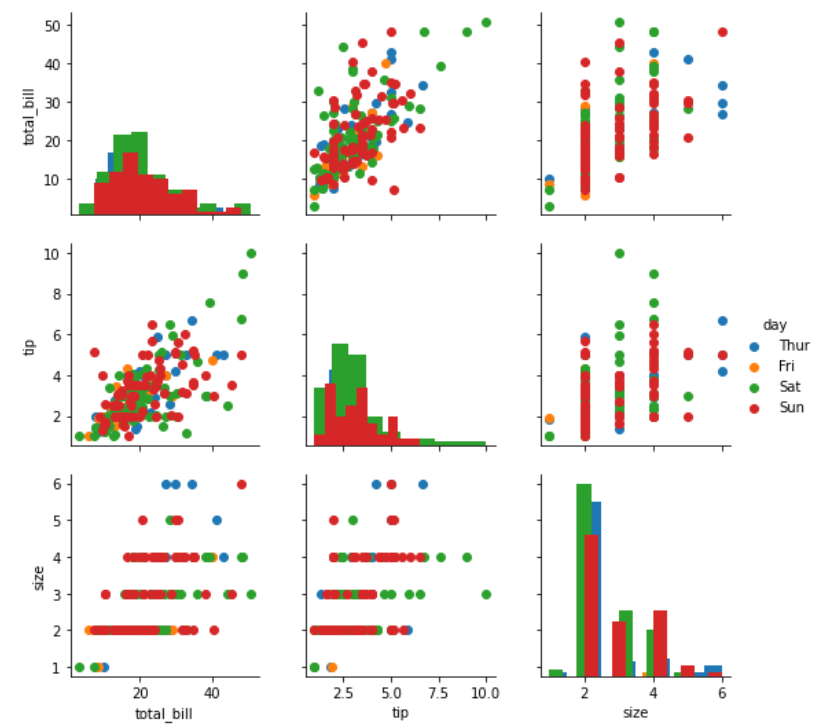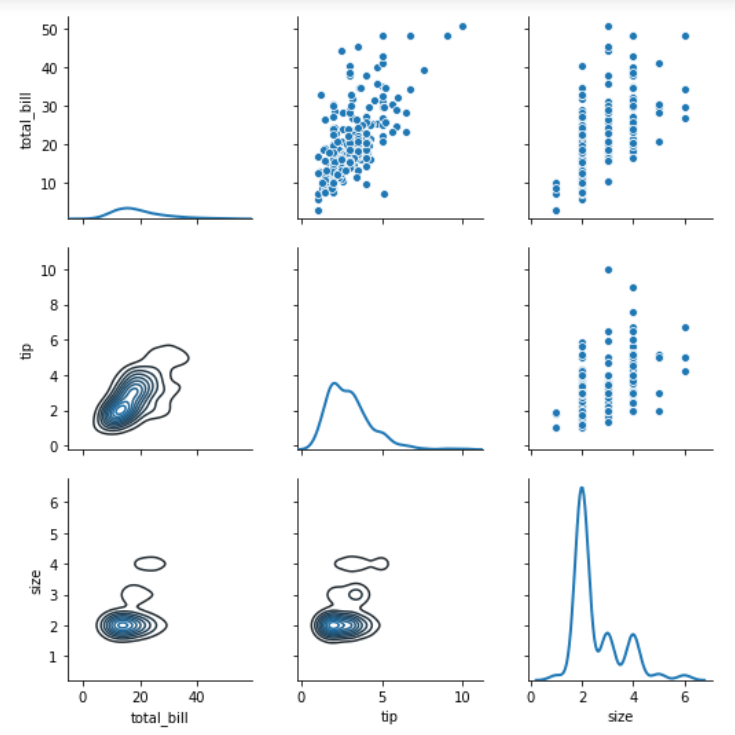Open In App
Related Articles
• Write an Interview Experience

# Python – seaborn.PairGrid() method

#### Prerequisite: Seaborn Programming Basics

Seaborn is a Python data visualization library based on matplotlib. It provides a high-level interface for drawing attractive and informative statistical graphics. Seaborn helps resolve the two major problems faced by Matplotlib; the problems are ?

• Default Matplotlib parameters
• Working with data frames

As Seaborn compliments and extends Matplotlib, the learning curve is quite gradual. If you know Matplotlib, you are already half way through Seaborn.

### seaborn.PairGrid() :

• Subplot grid for plotting pairwise relationships in a dataset.
• This class maps each variable in a dataset onto a column and row in a grid of multiple axes. Different axes-level plotting functions can be used to draw bivariate plots in the upper and lower triangles, and the marginal distribution of each variable can be shown on the diagonal.
• It can also represent an additional level of conditionalization with the hue parameter, which plots different subsets of data in different colors. This uses color to resolve elements on a third dimension, but only draws subsets on top of each other and will not tailor the hue parameter for the specific visualization the way that axes-level functions that accept hue will.
`                    seaborn.PairGrid( data, \*\*kwargs)`

Seaborn.PairGrid uses many arguments as input, main of which are described below in form of table:

Below is the implementation of above method:

Example 1:

## Python3

 `# importing packages``import` `seaborn``import` `matplotlib.pyplot as plt` `# loading dataset``df ``=` `seaborn.load_dataset(``'tips'``)` `# PairGrid object with hue``graph ``=` `seaborn.PairGrid(df, hue ``=``'day'``)``# type of graph for diagonal``graph ``=` `graph.map_diag(plt.hist)``# type of graph for non-diagonal``graph ``=` `graph.map_offdiag(plt.scatter)``# to add legends``graph ``=` `graph.add_legend()``# to show``plt.show()``# This code is contributed by Deepanshu Rusatgi.`

#### Output :## Python3

 `# importing packages``import` `seaborn``import` `matplotlib.pyplot as plt` `# loading dataset``df ``=` `seaborn.load_dataset(``'tips'``)` `# PairGrid object with hue``graph ``=` `seaborn.PairGrid(df)``# type of graph for non-diagonal(upper part)``graph ``=` `graph.map_upper(sns.scatterplot)``# type of graph for non-diagonal(lower part)``graph ``=` `graph.map_lower(sns.kdeplot)``# type of graph for diagonal``graph ``=` `graph.map_diag(sns.kdeplot, lw ``=` `2``)``# to show``plt.show()``# This code is contributed by Deepanshu Rusatgi.`

#### Output: